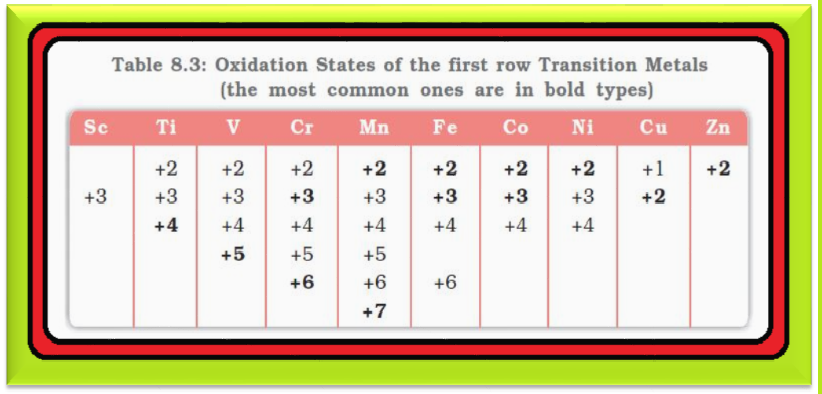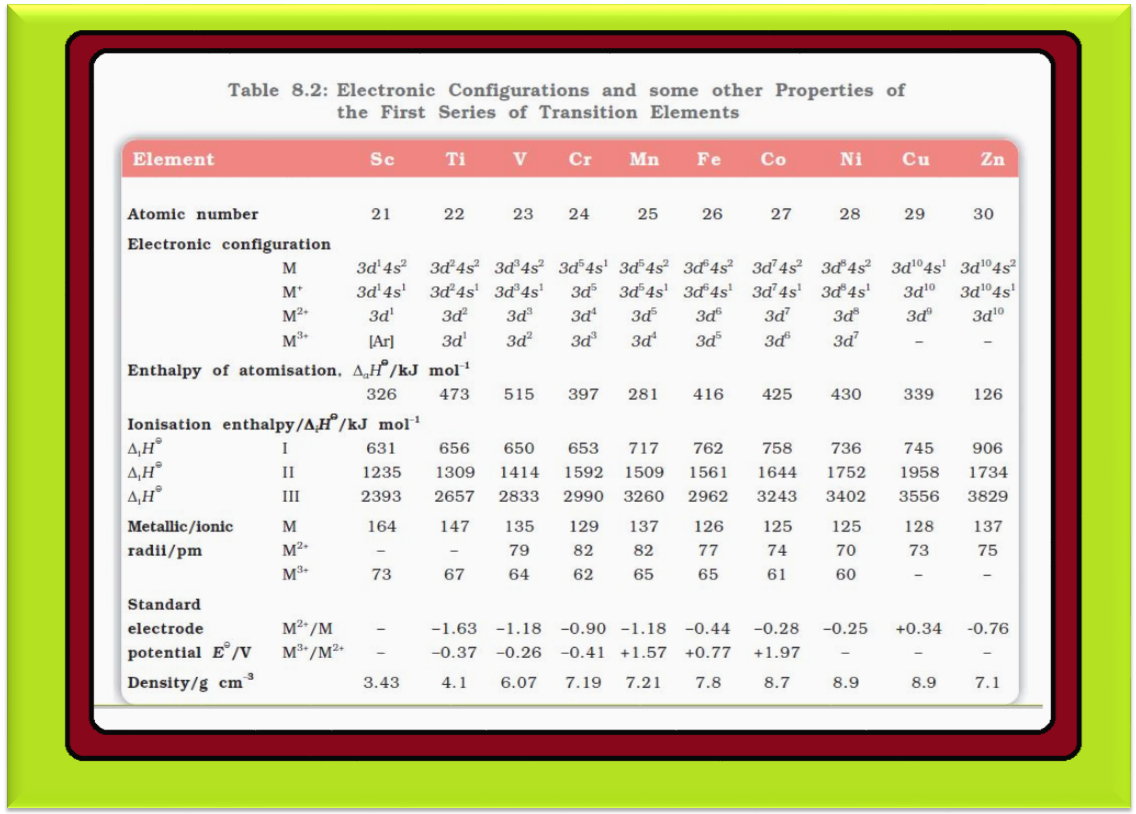Chemistry General Properties of the Transition Elements (d-Block)-2

### Topics Covered :

● Oxidation States
● Trends in the M^(+2)|M Standard Electrode Potential
● Trends in the M^(+3)|M^(+2) Standard Electrode Potential
● Trends in Stability of Higher Oxidation State

### Oxidation States :=> One of the notable features of a transition element is the great variety of oxidation states it may show in its compounds.

=> Table 8.3 lists the common oxidation states of the first row transition elements.

=> The elements which give the greatest number of oxidation states occur in or near the middle of the series.

● Manganese, for example, exhibits all the oxidation states from +2 to +7.

=> The lesser number of oxidation states at the extreme ends stems from either too few electrons to lose or share (color{red}(Sc), color{red}(Ti)) or too many color{red}(d) electrons (hence fewer orbitals available in which to share electrons with others) for higher valence (color{red}(Cu), color{red}(Zn)).

● Thus, early in the series scandium(II) is virtually unknown and titanium (IV) is more stable than color{red}(Ti )(III) or color{red}(Ti)(II).

● At the other end, the only oxidation state of zinc is +2 (no color{red}(d) electrons are involved).

● The maximum oxidation states of reasonable stability correspond in value to the sum of the s and d electrons upto manganese (color{red}(Ti^(IV)O_2), color{red}(V^(V)O_2^+), color{red}(Cr^(VI)O_4^(2-)), color{red}(Mn^(VII)O_4^-)) followed by a rather abrupt decrease in stability of higher oxidation states, so that the typical species to follow are color{red}(Fe^(II,III)), color{red}(Co^(II,III)), color{red}(Ni^(II)), color{red}(Cu^(I,II)), color{red}(Zn^(II)).

=> The variability of oxidation states, a characteristic of transition elements, arises out of incomplete filling of d orbitals in such a way that their oxidation states differ from each other by unity, e.g., color{red}(V^(II)), color{red}(V^(III)), color{red}(V^(IV)), color{red}(V^V).

● This is not in agreement with the variability of oxidation states of non transition elements where oxidation states normally differ by a unit of two.

=> An interesting feature in the variability of oxidation states of the color{red}(d)–block elements is noticed among the groups (groups 4 through 10).

color{red}(text(Note )) :  In the color{red}(p)–block the lower oxidation states are favoured by the heavier members (due to inert pair effect), the opposite is true in the groups of color{red}(d)-block.

● For example, in group 6, color{red}(Mo(VI)) and color{red}(W(VI)) are found to be more stable than color{red}(Cr(VI)). Thus color{red}(Cr(VI)) in the form of dichromate in acidic medium is a strong oxidising agent, whereas color{red}(MoO_3) and color{red}(WO_3) are not.

=> Low oxidation states are found when a complex compound has ligands capable of color{red}(π)-acceptor character in addition to the color{red}(σ)-bonding. For example, in color{red}(Ni(CO)_4) and color{red}(Fe(CO)_5), the oxidation state of nickel and iron is zero.
Q 3010191919Name a transition element which does not exhibit variable oxidation states.Solution:

Scandium (Z = 21) does not exhibit variable oxidation states .

### Trends in the M^(2+)|M Standard Electrode Potentials :=> Table 8.4 contains the thermochemical parameters related to the transformation of the solid metal atoms to color{red}(M^(2+)) ions in solution and their standard electrode potentials.

=> The observed values of color{red}(E^⊖) and those calculated using the data of Table 8.4 are compared in Fig. 8.4.

=> The unique behaviour of color{red}(Cu), having a positive color{red}(E^⊖), accounts for its inability to liberate color{red}(H_2) from acids.

=> Only oxidising acids (nitric and hot concentrated sulphuric) react with color{red}(Cu), the acids being reduced.

=> The high energy to transform color{red}(Cu(s)) to color{red}(Cu^(2+))(aq) is not balanced by its hydration enthalpy.

=> The general trend towards less negative color{red}(E^⊖) values across the series is related to the general increase in the sum of the first and second ionisation enthalpies.

color{red}(text(Note )) : The value of color{red}(E^(⊖)) for color{red}(Mn), color{red}(Ni) and color{red}(Zn) are more negative than expected from the trend.

Q 3031101022Why is Cr^(2+) reducing and Mn^(3+) oxidising when both have d^4 configuration.Solution:

Cr^(2+) is reducing as its configuration changes from d^4 to d^3, the latter having a half-filled t_(2g) level (see Unit 9) . On the other hand, the change from Mn^(2+) to Mn^(3+) results in the half-filled (d^5) configuration which has extra stability.

### Trends in the M^(3+)|M^(2+) Standard Electrode Potentials :=> An examination of the color{red}(E (M^(3+) | M^(2+) )) values (Table 8.2) shows the varying trends.

=> The low value for color{red}(Sc) reflects the stability of color{red}(Sc^(3+)) which has a noble gas configuration.

=> The highest value for color{red}(Zn) is due to the removal of an electron from the stable color{red}(d^(10)) configuration of color{red}(Zn^(2+)).

=> The comparatively high value for color{red}(Mn) shows that color{red}(Mn^(2+) (d^5)) is particularly stable, whereas comparatively low value for color{red}(Fe) shows the extra stability of color{red}(Fe^(3+) (d^5)).

=> The comparatively low value for color{red}(V) is related to the stability of color{red}(V^(2+)) (half-filled color{red}(t_(2g)) level).

### Trends in Stability of Higher Oxidation States :=> Table 8.5 shows the stable halides of the color{red}(3d) series of transition metals.

=> The highest oxidation numbers are achieved in color{red}(TiX_4) (tetrahalides), color{red}(VF_5) and color{red}(CrF_6).

=> The +7 state for color{red}(Mn) is not represented in simple halides but color{red}(MnO_3F) is known, and beyond color{red}(Mn) no metal has a trihalide except color{red}(FeX_3) and color{red}(CoF_3).

=> The ability of fluorine to stabilise the highest oxidation state is due to either higher lattice energy as in the case of color{red}(CoF_3), or higher bond enthalpy terms for the higher covalent compounds, e.g., color{red}(VF_5) and color{red}(CrF_6).

● color{red}(V^V) is represented only by color{red}(VF_5), the other halides, however, undergo hydrolysis to give oxohalides, color{red}(VOX_3).

● Another feature of fluorides is their instability in the low oxidation states e.g., color{red}(VX_2) (color{red}(X = CI), color{red}(Br) or color{red}(I)) and the same applies to color{red}(CuX).

=> All color{red}(Cu^I) halides are known except the iodide. In this case, color{red}(Cu^(2+)) oxidises color{red}(I^-) to color{red}(I_2) :

color{red}(2Cu^(2-) + 4I^(-) -> Cu_2 I_2 (s) + I_2)

=> But, many copper (I) compounds are unstable in aqueous solution and undergo disproportionation.

color{red}(2Cu^(+) → Cu^(2+) + Cu)

● The stability of color{red}(Cu^(2+)) (aq) rather than color{red}(Cu^(+)) (aq) is due to the much more negative color{red}(Δ_(hyd)H^⊖) of color{red}(Cu^(2+)) (aq) than color{red}(Cu^(+)), which more than compensates for the second ionisation enthalpy of color{red}(Cu).

=> The ability of oxygen to stabilise the highest oxidation state is demonstrated in the oxides.

● The highest oxidation number in the oxides (Table 8.6) coincides with the group number and is attained in color{red}(Sc_2O_3) to color{red}(Mn_2O_7).

● Beyond Group 7, no higher oxides of color{red}(Fe) above color{red}(Fe_2O_3), are known, although ferrates (VI) color{red}((FeO_4)^(2–)), are formed in alkaline media but they readily decompose to color{red}(Fe_2O_3) and color{red}(O_2).

● Besides the oxides, oxocations stabilise color{red}(V^v) as color{red}(VO_2^+), color{red}(V^(IV)) as color{red}(VO^(2+)) and color{red}(Ti^(IV)) as color{red}(TiO^(2+)).

=> The ability of oxygen to stabilise these high oxidation states exceeds that of fluorine. Thus, the highest color{red}(Mn) fluoride is color{red}(MnF_4) whereas the highest oxide is color{red}(Mn_2O_7).

=> The ability of oxygen to form multiple bonds to metals explains its superiority.

=> In the covalent oxide color{red}(Mn_2O_7), each color{red}(Mn) is tetrahedrally surrounded by color{red}(O’s) including a color{red}(Mn–O–Mn) bridge.

=> The tetrahedral color{red}([MO_4]^n) ions are known for color{red}(V^V), color{red}(Cr^(Vl)), color{red}(Mn^(V)), color{red}(Mn^(Vl)) and color{red}(Mn^(VII)).
Q 3061101025How would you account for the increasing oxidising power in the series VO^(2+) < Cr_2O_7^(2–) < MnO_4^(
–) ?Solution:

This is due to the increasing stability of the lower species to which they are reduced.